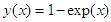﻿ A 4-Point Block Method for Solving Second Order Initial Value Problems in Ordinary Differential EquationsAmerican Journal of Computational and Applied Mathematics

p-ISSN: 2165-8935    e-ISSN: 2165-8943

2019;  9(3): 51-56

doi:10.5923/j.ajcam.20190903.01### A 4-Point Block Method for Solving Second Order Initial Value Problems in Ordinary Differential Equations

L. A. Ukpebor

Department of Mathematics, Ambrose Alli University, Ekpoma, Nigeria

Correspondence to: L. A. Ukpebor, Department of Mathematics, Ambrose Alli University, Ekpoma, Nigeria.
 Email: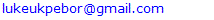Abstract

This paper proposes a four-point block method for the numerical solution of second order ordinary differential equations. In the construction of the method, interpolation and collocation techniques were involved, while Legendre Polynomial served as both the interpolation and collocation equations. The proposed scheme was analyzed and it was found to be A-stable, consistent and convergent.Numerical examples of ordinary differential equations were solved using the proposed method to test its performance. The results obtained compared favourably with other methods considered.

Keywords: Interpolation, Collocation, Block method, Legendre Polynomial, A-stable, Consistent, Convergent

Cite this paper: L. A. Ukpebor, A 4-Point Block Method for Solving Second Order Initial Value Problems in Ordinary Differential Equations, American Journal of Computational and Applied Mathematics , Vol. 9 No. 3, 2019, pp. 51-56. doi: 10.5923/j.ajcam.20190903.01.

### 1. Introduction

This paper considered the general second-order ordinary differential equations, ODEs which we often encounter in the field of sciences, engineering and dynamic systems. They are generally written as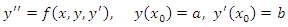(1)
My intention in this paper is to derive a block scheme from the continuous linear multistep method of the form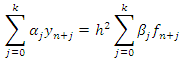(2)
that can solve (1) above. Some authors have attempted solving (1) numerically by first reducing it to a system of first order equations. Notable among these authors are  and . In spite of the success of this method, it suffers some setbacks. According to , the method is un-economical in terms of cost of implementation, increased computational burden and wastage of computer time and increased dimension of the resulting systems of equations to be solved.
Some researchers have attempted solving (1) directly without reducing it to a first order ODEs by employing continuous linear multistep methods. See ,  and . According to , continuous linear multistep method have greater advantages over the discrete methods since they give better error estimates and provide simplified form, which allows easy approximation of solution at all interior points of the integration interval.
Using linear multistep methods, several other researchers [7-17], have proposed methods in which the approximate solutions range from Power Series, Chebychev’s, Lagrange’s and Laguerre’s polynomials.
In this paper, Legendre polynomial was used as basis function in generating the continuous linear multistep for the solution of (1).
The plan of this paper is as follows: Section 1, introduction; Section 2, the derivation of the proposed method is presented. In Section 3, the stability and convergence analysis of the block method is given. In Section 4, numerical examples are considered. The paper ends with summary and conclusion in Section 5.

### 2. Materials and Methods

In this section, the mathematical formulation of the method is presented. I use Legendre polynomial as our approximate solution.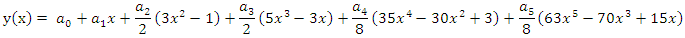(3)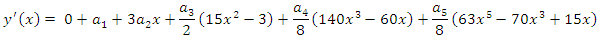(4)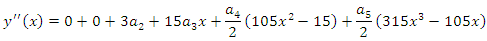(5)
Interpolating (3) at the points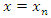and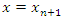, we have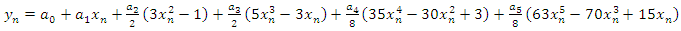(6)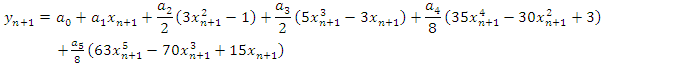(7)
Also, collocating (5) at the points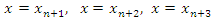and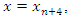we have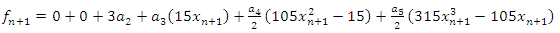(8)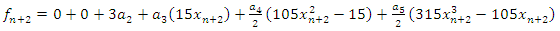(9)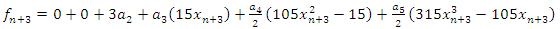(10)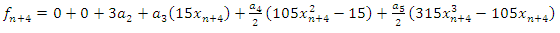(11)
Putting (6-11) in matrix form gives below D matrix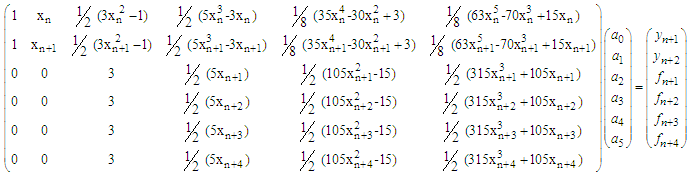(12)
Solving (12) for the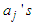and substituting back into (3) and after much algebraic simplification, to obtained the multistep method of the form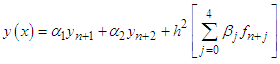(13)
Where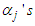and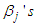are continuous coefficients expressed as functions of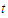, where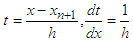(14)
The coefficients of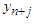and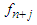are obtained as: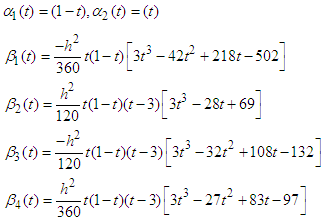(15)
Evaluating (15) at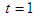, the first derivatives of (15) at all the points and solve simultaneously gives the numerical integrators below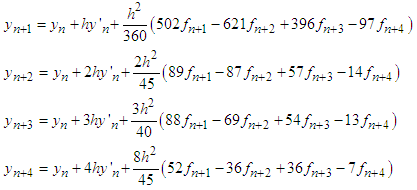(16)
With the following derivatives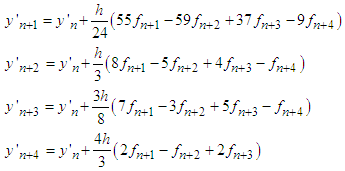(17)
(16) and (17) are the required formulae for the implementation of the method.

### 3. Convergence Analysis of (OEBHF)

#### 3.1. Order and Error Constants of the New Method

According to ([1, 16, 17]), the order of the new method in Equation (11) is obtained by using the Taylor series and it is found that the developed method has uniformly order four, with an error constants vector of: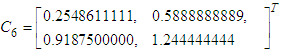#### 3.2. Consistency

Definition 3.1: The hybrid block method (7) is said to be consistent if it has an order more than or equal to one i.e.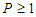. Therefore, the method is consistent ([1, 4, 6]).

#### 3.3. Regions of Absolute Stability (RAS)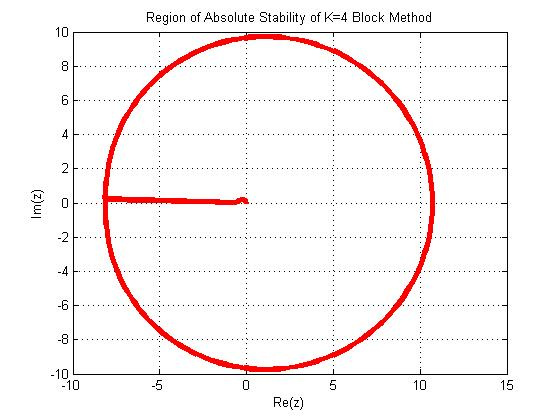Figure 1. Absolute Stability Region of the new method

### 4. Numerical Implementation of the New Method

In this section, the accuracies and the performance the new developed methods is tested on two standard test problems from the recent literature. Two numerical experiments widely solved by Kayode et al (2018), and Kayode and Adeyeye (2013). The performance of the new developed method is shown in Tables 1-5, while table 6-7 shows the comparison of the new developed method with the selected problem in the existing method Kayode et al (2018), and Kayode and Adeyeye (2013) in terms of absolute errors and accuracies.
 Table 1. Results of Problem 1 with h=0.1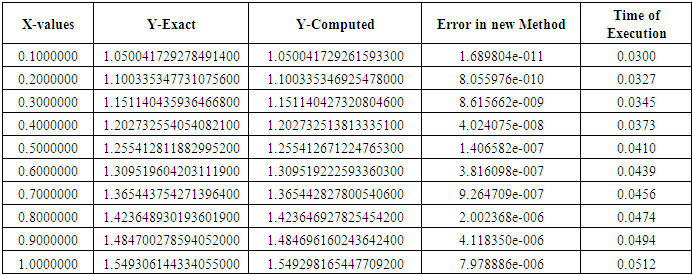Table 2. Results of Problem 1 with h=0.01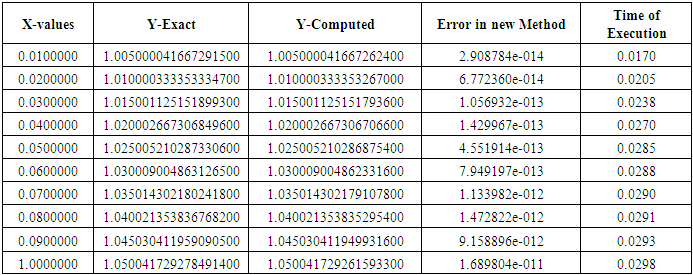Table 3. Results of Problem 1 with h=0.1/32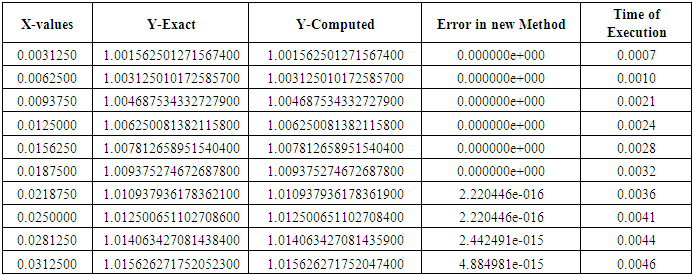Table 4. Results of Problem 2 with h =0.1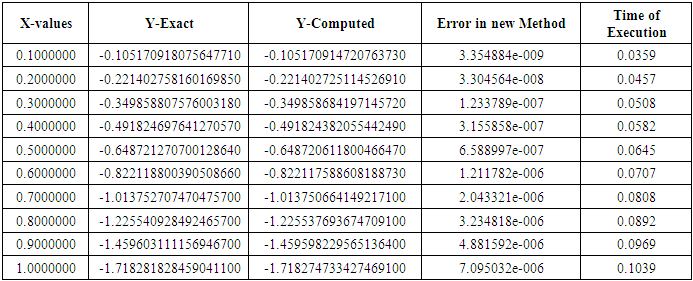Table 5. Results of Problem 2 with h =0.1/32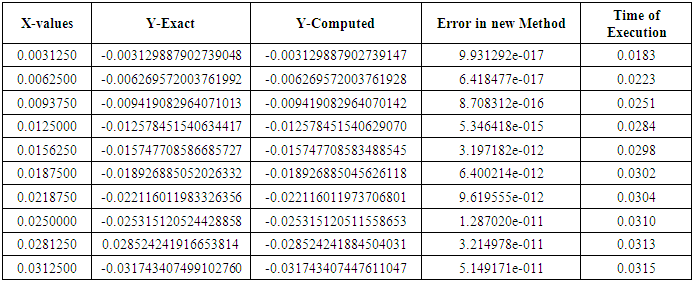Table 6. Comparison of Problem 1 with Kayode et al (2018)  with h =0.1/32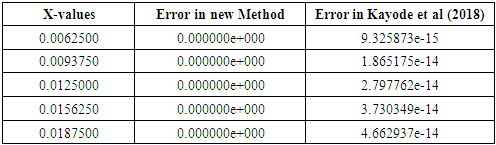Table 7. Comparison of Problem 2 with Kayode and Adeyeye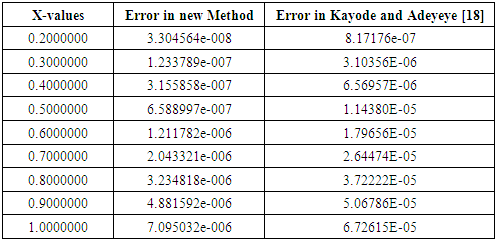Problem I: Considered a non-linear second order ordinary differential equations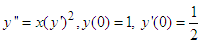Exact solution: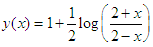Source: Kayode et al (2018)
Problem II: Considered a linear second order ordinary differential equations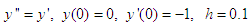Exact solution